Copyright (C) 2012-present, The Computational Data Science Lab
https://github.com/cdslaborg/paramonte

# Making 3D kernel-density-estimate contour plots with the ParaMonte visualization tools

NOTE
If you are viewing an HTML version of this MATLAB live script on the web, you can download the corresponding MATLAB live script visualization_contour3.mlx file to this HTML page at,
https://github.com/cdslaborg/paramontex/tree/main/MATLAB/mlx
Once you download the file, open it in MATLAB to view and interact with its contents, which is the same as what you see on this page.
First, let's clean up the MATLAB environment and make sure the path to the ParaMonte library is in MATLAB's path list.
clc;
clear all;
close all;
format compact; format long;
%%%%%%%%%%%% IMPORTANT %%%%%%%%%%%%%
% Set the path to the ParaMonte library:
% Change the following path to the ParaMonte library root directory,
% otherwise, make sure the path to the ParaMonte library is already added
% to MATLAB's path list.
pmlibRootDir = './';
%%%%%%%%%%%%%%%%%%%%%%%%%%%%%%%%%%%%
% change MATLAB's working directory to the folder containing this script
% if MATLAB Live Scripts did not create a temporary folder, we would not
% have all of these problems!
try
setwdFilePath = websave("setwd.m","https://github.com/cdslaborg/paramontex/raw/main/MATLAB/mlx/setwd.m");
run(setwdFilePath); % This is a MATLAB script that you can download from the same GitHub location given in the above.
catch % alas, we will have to run the simulations in MATLAB Live Script's temporary folder
filePath = mfilename('fullpath');
[currentDir,fileName,fileExt] = fileparts(filePath); cd(currentDir);
cd(fileparts(mfilename('fullpath'))); % Change working directory to source code directory.
end

## ParaMonte's default visualization tools

The ParaMonte library ships with several visualization tools that automate much of the MATLAB coding required to visualize the output of the simulations performed by the ParMonte library samplers.
By replacing the input dataFrame to these tools and following the conventions of the ParaMonte library, one can also use these visualization tools for any dataset that may not have been generated by the ParaMonte library.
Consider the following Markov chain on the web in compact format generated by the ParaDRAM sampler of the ParaMonte library to sample a MultiVariate Normal distribution. Since this is a chain file as inidicated by its suffix "_chain.txt" We will read this file via the ParaDRAM sampler's readChain() method.
pm = paramonte();
This method automatically generates a set of tools that can be used to visualize the contents of the compact chain file. Note that these visualization tools are not unique to this particular method of the ParaDRAM sampler or other ParaMonte samplers. For the sake of illustration however, we will create plots using the above dataset read via readChain() method of the ParaDRAM routine.
chain = pmpd.chainList{1};
chain.plot.contour3.make();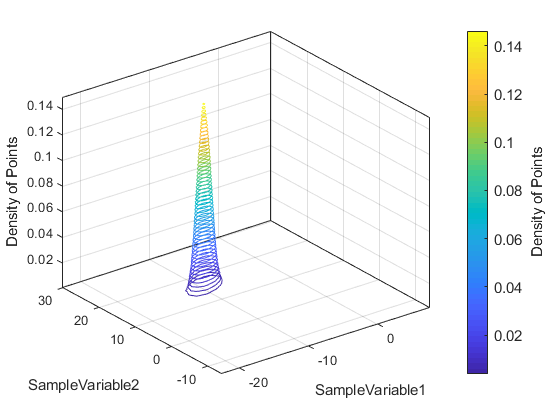Be default, the visualization tools are loaded with a set of predefined settings. For example, ParaMonte visualizations are by default colored (unless mutiple variables are to be displayed). These however, can be readily changed. For example, to change the colormap,
chain.plot.contour3.colormap
ans = struct with fields:
enabled: 1 values: "parula"
chain.plot.contour3.colormap.values = autumn;
chain.plot.contour3.make()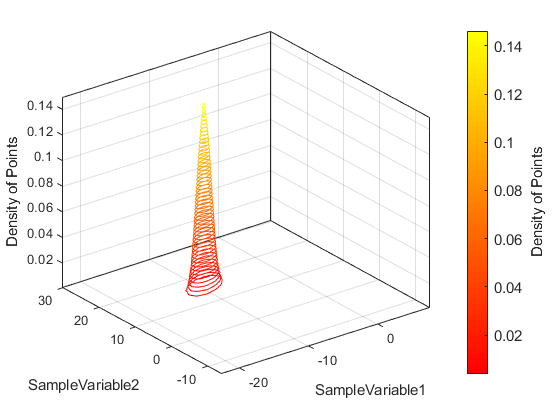To draw the 3D kernel density estimate plots, the ParaMonte visualizer utilizes the contour3() function of MATLAB. One can pass pairs of (key,value) properties to this MATLAB function by defining those keyword properties in the contour3 component of the plot object. There are a few properties defined already in this structure,
chain.plot.contour3.contour3.kws
ans = struct with fields:
color: [] lineStyle: "-" lineWidth: 0.500000000000000 showText: "off" labelSpacing: 144
chain.plot.contour3.colormap.values = flipud(cold());
chain.plot.contour3.make();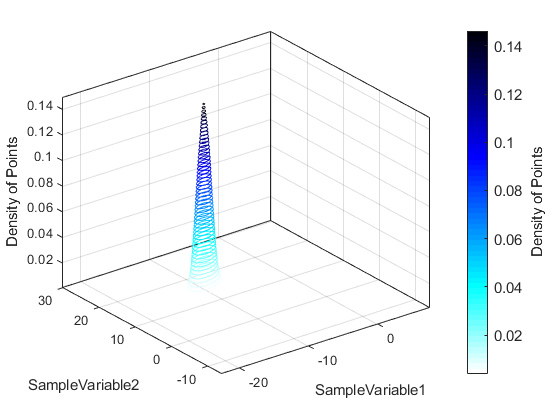To reset the properties of the plot object to the default settings, try,
chain.plot.contour3.reset();
ParaDRAM - NOTE: resetting the properties of the contour3 plot...
To reset the entire plot object including reading the data again from the input dataFrame, try,
chain.plot.contour3.reset("hard");
ParaDRAM - NOTE: creating the contour3 plot object from scratch...
Similarly, to change the properties of the colorbar, try,
chain.plot.contour3.colorbar.kws
ans = struct with fields: fontSize: []
chain.plot.contour3.colorbar.kws.fontSize = 12;
To change properties that do not exist, simple add them to the kws component, for example,
chain.plot.contour3.colorbar.kws.location = "northoutside";
chain.plot.contour3.make();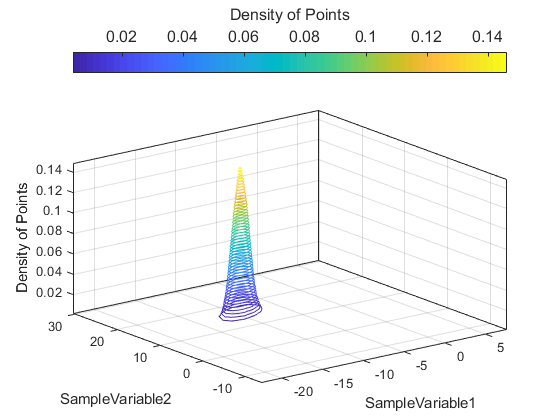chain.plot.contour3.colorbar.kws
ans = struct with fields:
fontSize: 12 location: "northoutside"
Remember that a handle to all objects in the plot is also stored in the currentFig component of the object. Most of the properties of the figure, axes, and the plots can be also changed directly via these handles. For example, to change the colorbar label, we could try,
chain.plot.contour3.currentFig.colorbar.Label.String
ans = 'Density of Points'
chain.plot.contour3.reset();
ParaDRAM - NOTE: resetting the properties of the contour3 plot...
chain.plot.contour3.make();
chain.plot.contour3.currentFig.axes.ZScale = "log";
chain.plot.contour3.currentFig.colorbar.Label.FontSize = 12;
chain.plot.contour3.currentFig.colorbar.Label.Interpreter = "tex"; % set the interpreter for the colorbar
chain.plot.contour3.currentFig.colorbar.Label.String = "Density of Sampled Points";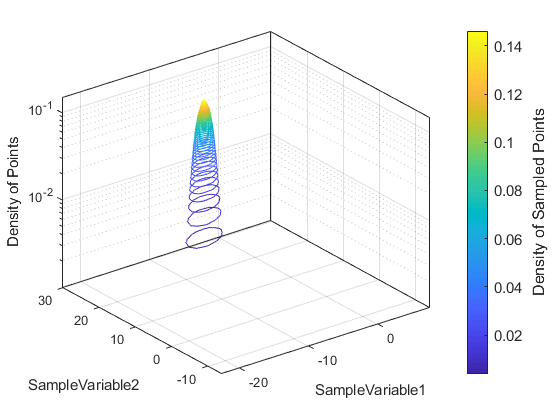## Setting the resolution and the noise tolerance of the plot

If the resolution of the kernel density estimate in the plot is low, it can be increased by changing the value of the gridSize component of the plot object,
chain.plot.contour3.gridSize
ans = 512
This number must be a power of two (otherwise it will be made so, automatically). It represents the number of grid points along each axis of the plot and is used to set the resolution of the kernel density estimate. The higher this number, the higher the resolution of the estimate will be. However, larger values will be also computationally more expensive.
Similarly, if the resulting kernel density estimate appears to be noisy in the background, or perhaps there are some small density variations far from the high-density region that we may not want to display in the plot, we could remove it via,
chain.plot.contour3.noiseLevel
ans = 1.000000000000000e-03
Any density value below this threshold will be set to nan, such that it will not be displayed in the plot.

## Choosing different columns of data to plot

By default, the column named the first two variables of the sampled space are shown in the plot. This can be readily changed to any paris of variables, like,
chain.df.Properties.VariableNames
ans = 1×11 cell array {'ProcessID'} {'DelayedRejectionStage'} {'MeanAcceptanceRate'} {'AdaptationMeasure'} {'BurninLocation'} {'SampleWeight'} {'SampleLogFunc'} {'SampleVariable1'} {'SampleVariable2'} {'SampleVariable3'} {'SampleVariable4'}
chain.plot.contour3.make( "xcolumns", "SampleVariable3", "ycolumns", 11);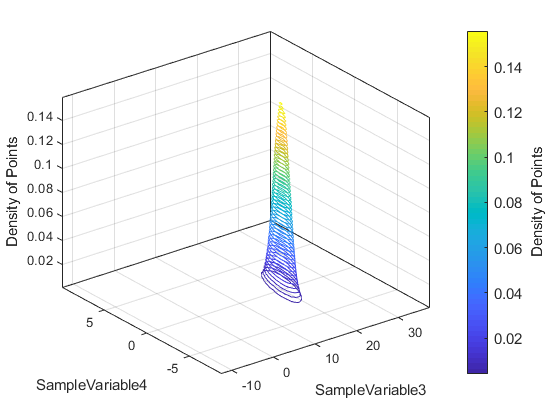Notice the possibility of use of both column indices and column names to point to a data column in the dataFrame.

## Unicolor plot

Turning the colormap off is very simple,
chain.plot.contour3.colormap.enabled = false; % make monocolor plot
chain.plot.contour3.make();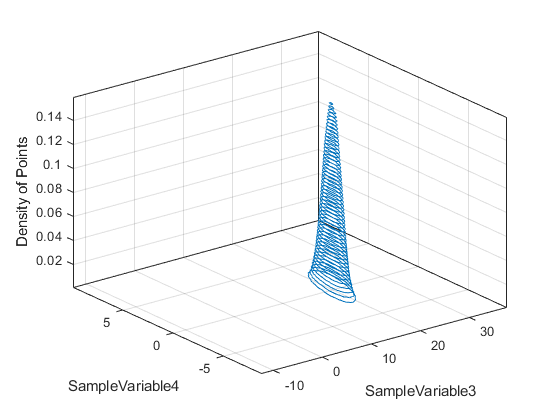## Plotting multiple columns of data in a single plot

The columns of data that are plotted are determined by the corresponding column names in xcolumns and ycolumns of the plot object:
• If xcolumns is set to empty [], then the count of data will be used as the x values,
• If ycolumns is set to empty [], then the all data columns will be drawn on the same plot (not recommended),
chain.df.Properties.VariableNames
ans = 1×11 cell array {'ProcessID'} {'DelayedRejectionStage'} {'MeanAcceptanceRate'} {'AdaptationMeasure'} {'BurninLocation'} {'SampleWeight'} {'SampleLogFunc'} {'SampleVariable1'} {'SampleVariable2'} {'SampleVariable3'} {'SampleVariable4'}
chain.plot.contour3.xcolumns
ans = "SampleVariable3"
chain.plot.contour3.ycolumns
ans = 11
To make plots multiple columns of data in a single plot, simply add the column names to the corresponding component, or more simply,
chain.plot.contour3.reset("hard");
ParaDRAM - NOTE: creating the contour3 plot object from scratch...
chain.plot.contour3.colormap.enabled = false;
chain.plot.contour3.xcolumns = 8:9;
chain.plot.contour3.legend.enabled = true;
chain.plot.contour3.legend.labels = ["variable1 - variable4", "variable2 - variable3"];
chain.plot.contour3.legend.kws.location = "northwest";
chain.plot.contour3.make("ycolumns", ["SampleVariable4", 10]) % notice the ability to mix column number with column names, or simply pass column ranges
view([46 54]); % change the 3D view to this angle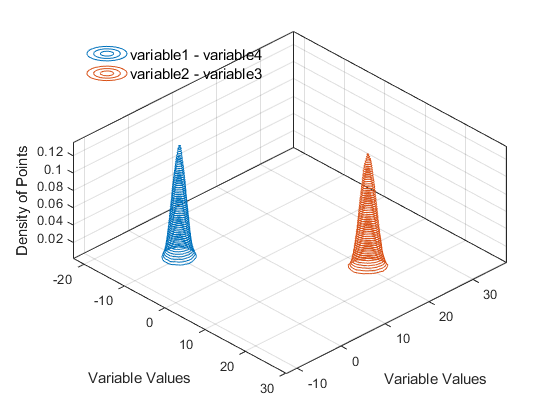or, multiple variables against a single variable,
chain.plot.contour3.reset("hard");
ParaDRAM - NOTE: creating the contour3 plot object from scratch...
chain.plot.contour3.colormap.enabled = false;
chain.plot.contour3.legend.enabled = true;
chain.plot.contour3.legend.kws.location = "northwest";
chain.plot.contour3.legend.labels = []; % reset the labels to automatic
chain.plot.contour3.make("xcolumns", "SampleVariable2", "ycolumns", [10,11])